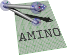amino  1.0-beta2 Lightweight Robot Utility Library
type.h File Reference

Types for transforms. More...

Go to the source code of this file.

## Classes

struct  aa_tf_rotmatp
Memory layout for a planar rotation matrix. More...

struct  aa_tf_cv
Memory layout for planar transform as complex number and translation vector. More...

struct  aa_tf_tfmatp
Memory layout for a planar transformation matrix. More...

struct  aa_tf_vec3
Memory layout for a vector of length 3. More...

struct  aa_tf_rotmat
Memory layout for a rotation matrix. More...

struct  aa_tf_axang
Memory layout for axis-Angle rotation in x,y,z,angle order. More...

struct  aa_tf_quat
Memory layout for a quaternion, x,y,z,w order. More...

struct  aa_tf_eulerzyx
Memory layout for an Euler Angle in ZYX (yaw-pitch-roll) format. More...

struct  aa_tf_tfmat
Memory layout for a transformation matrix. More...

struct  aa_tf_qv
Memory layout for a Transformation as rotation quaternion and translation vector. More...

struct  aa_tf_duqu
Memory layout for a dual quaternion. More...

struct  aa_tf_dx
Memory layout for an SE(3) velocity. More...

struct  aa_tf_qv_dx
Transform and spatial velocity. More...

## Macros

#define AA_TF_X   0
Index of vector x element.

#define AA_TF_Y   1
Index of vector y element.

#define AA_TF_Z   2
Index of vector z element.

#define AA_TF_QUAT_V   0
Index of quaternion vector part.

#define AA_TF_QUAT_XYZ   AA_TF_QUAT_V
Index of quaternion vector part.

#define AA_TF_QUAT_X   (AA_TF_QUAT_V + AA_TF_X)
Index of quaternion vector x.

#define AA_TF_QUAT_Y   (AA_TF_QUAT_V + AA_TF_Y)
Index of quaternion vector y.

#define AA_TF_QUAT_Z   (AA_TF_QUAT_V + AA_TF_Z)
Index of quaternion vector z.

#define AA_TF_QUAT_W   3
Index of quaternion scalar part.

#define AA_TF_TFMAT_R   0
Index of rotation matrix part of transformation matrix.

#define AA_TF_TFMAT_V   9
Index of origin part of transformation matrix.

#define AA_TF_TFMAT_X   9
Index of origin x component of transformation matrix.

#define AA_TF_TFMAT_Y   10
Index of origin y component of transformation matrix.

#define AA_TF_TFMAT_Z   11
Index of origin z component of transformation matrix.

#define AA_TF_DX_V   0
Index of spatial velocity translational part.

#define AA_TF_DX_W   3
Index of spatial velocity rotational part.

#define AA_TF_QUTR_Q   0
Index of quaternion-translation quaternion part.

#define AA_TF_QUTR_QX   (AA_TF_QUTR_Q + AA_TF_QUAT_X)
Index of quaternion-translation quaternion x.

#define AA_TF_QUTR_QY   (AA_TF_QUTR_Q + AA_TF_QUAT_Y)
Index of quaternion-translation quaternion y.

#define AA_TF_QUTR_QZ   (AA_TF_QUTR_Q + AA_TF_QUAT_Z)
Index of quaternion-translation quaternion z.

#define AA_TF_QUTR_QW   (AA_TF_QUTR_Q + AA_TF_QUAT_W)
Index of quaternion-translation quaternion w.

#define AA_TF_QUTR_T   4
Index of quaternion-translation translation part.

#define AA_TF_QUTR_TX   (AA_TF_QUTR_T + AA_TF_X)
Index of quaternion-translation translation x.

#define AA_TF_QUTR_TY   (AA_TF_QUTR_T + AA_TF_Y)
Index of quaternion-translation translation y.

#define AA_TF_QUTR_TZ   (AA_TF_QUTR_T + AA_TF_Z)
Index of quaternion-translation translation z.

#define AA_TF_DUQU_REAL   0
index of dual quaternion real part

#define AA_TF_DUQU_DUAL   4
index of dual quaternion dual part

#define AA_TF_DUQU_REAL_W   (AA_TF_DUQU_REAL + AA_TF_QUAT_W)
index of dual quaternion real w

#define AA_TF_DUQU_REAL_XYZ   (AA_TF_DUQU_REAL + AA_TF_QUAT_XYZ)
index of dual quaternion real xyz

#define AA_TF_DUQU_REAL_X   (AA_TF_DUQU_REAL + AA_TF_QUAT_X)
index of dual quaternion real x

#define AA_TF_DUQU_REAL_Y   (AA_TF_DUQU_REAL + AA_TF_QUAT_Y)
index of dual quaternion real y

#define AA_TF_DUQU_REAL_Z   (AA_TF_DUQU_REAL + AA_TF_QUAT_Z)
index of dual quaternion real z

#define AA_TF_DUQU_DUAL_W   (AA_TF_DUQU_DUAL + AA_TF_QUAT_W)
index of dual quaternion dual w

#define AA_TF_DUQU_DUAL_XYZ   (AA_TF_DUQU_DUAL + AA_TF_QUAT_XYZ)
index of dual quaternion dual xyz

#define AA_TF_DUQU_DUAL_X   (AA_TF_DUQU_DUAL + AA_TF_QUAT_X)
index of dual quaternion dual x

#define AA_TF_DUQU_DUAL_Y   (AA_TF_DUQU_DUAL + AA_TF_QUAT_Y)
index of dual quaternion dual y

#define AA_TF_DUQU_DUAL_Z   (AA_TF_DUQU_DUAL + AA_TF_QUAT_Z)
index of dual quaternion dual z

## Typedefs

typedef double _Complex aa_tf_cmplx
Typedef for C99 complex numbers.

typedef aa_tf_cmplx aa_tf_vec2
Represent 2D vectors as complex numbers.

typedef struct aa_tf_rotmatp aa_tf_rotmatp
Memory layout for a planar rotation matrix. More...

typedef struct aa_tf_cv aa_tf_cv
Memory layout for planar transform as complex number and translation vector.

typedef struct aa_tf_tfmatp aa_tf_tfmatp
Memory layout for a planar transformation matrix. More...

typedef struct aa_tf_vec3 aa_tf_vec3_t
Memory layout for a vector of length 3.

typedef struct aa_tf_rotmat aa_tf_rotmat_t
Memory layout for a rotation matrix. More...

typedef struct aa_tf_axang aa_tf_axang_t
Memory layout for axis-Angle rotation in x,y,z,angle order. More...

typedef struct aa_tf_quat aa_tf_quat_t
Memory layout for a quaternion, x,y,z,w order. More...

typedef struct aa_tf_eulerzyx aa_tf_eulerzyx_t
Memory layout for an Euler Angle in ZYX (yaw-pitch-roll) format.

typedef struct aa_tf_tfmat aa_tf_tfmat_t
Memory layout for a transformation matrix. More...

typedef struct aa_tf_qv aa_tf_qv_t
Memory layout for a Transformation as rotation quaternion and translation vector.

typedef struct aa_tf_duqu aa_tf_duqu_t
Memory layout for a dual quaternion.

## Detailed Description

Types for transforms.

All types passed by reference are contiguous sequences of double floats and may safely be passed with a double* pointer to the first element. The structs in this file specify the memory layouts.

Complex numbers and 2D vectors are represented with C99 complex numbers and are passed and returned by value.

Definition in file type.h.

## ◆ aa_tf_axang_t

 typedef struct aa_tf_axang aa_tf_axang_t

Memory layout for axis-Angle rotation in x,y,z,angle order.

The first three elements are the axis x,y,z coordinates. The final element is the angle.

## ◆ aa_tf_quat_t

 typedef struct aa_tf_quat aa_tf_quat_t

Memory layout for a quaternion, x,y,z,w order.

The first three elements are the vector (x,y,z). The last element is the scalar (w).

## ◆ aa_tf_rotmat_t

 typedef struct aa_tf_rotmat aa_tf_rotmat_t

Memory layout for a rotation matrix.

column major

The matrix is in column-major order.

## ◆ aa_tf_rotmatp

 typedef struct aa_tf_rotmatp aa_tf_rotmatp

Memory layout for a planar rotation matrix.

Column major order.

## ◆ aa_tf_tfmat_t

 typedef struct aa_tf_tfmat aa_tf_tfmat_t

Memory layout for a transformation matrix.

It is a column-major matrix, but the bottom row is omitted because this row is the same for all SE(3) transformation matrices.

The first 9 elements are a column major rotation matrix. The last 3 elements are the origin vector.

## ◆ aa_tf_tfmatp

 typedef struct aa_tf_tfmatp aa_tf_tfmatp

Memory layout for a planar transformation matrix.

It is a column-major matrix, but the bottom row is omitted because this row is the same for all SE(2) transformation matrices.

The first 4 elements are a column major rotation matrix. The last 2 elements are the origin vector.